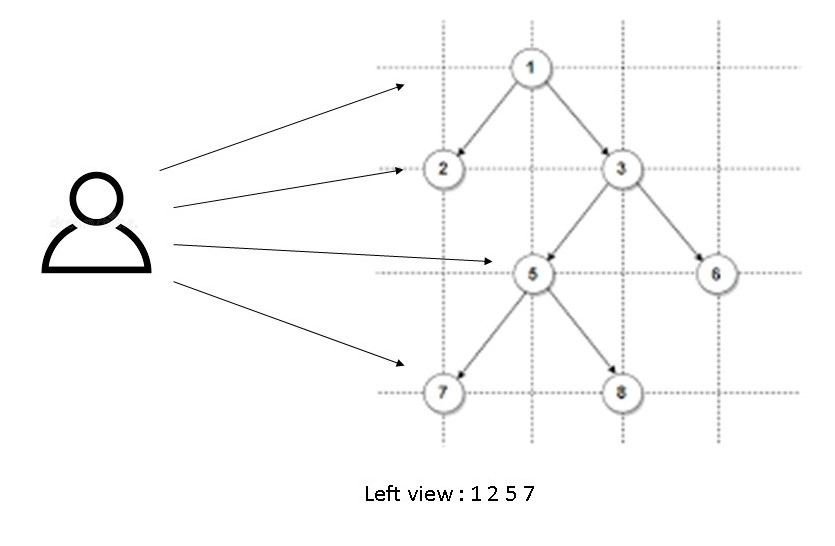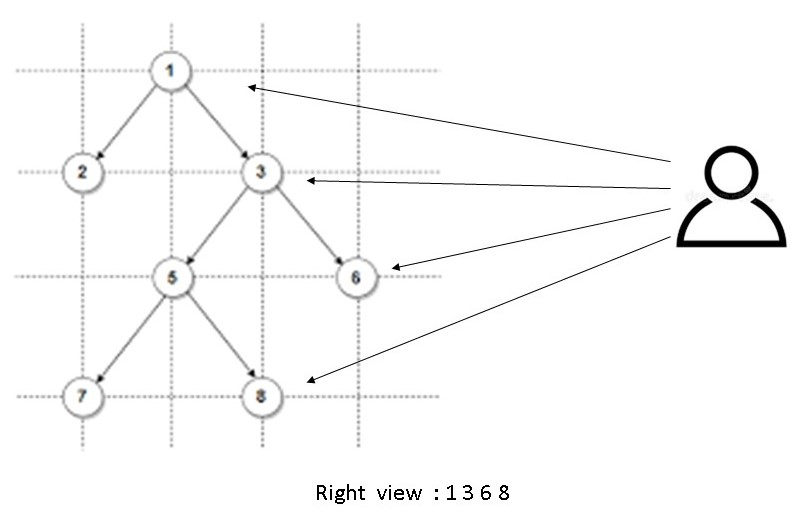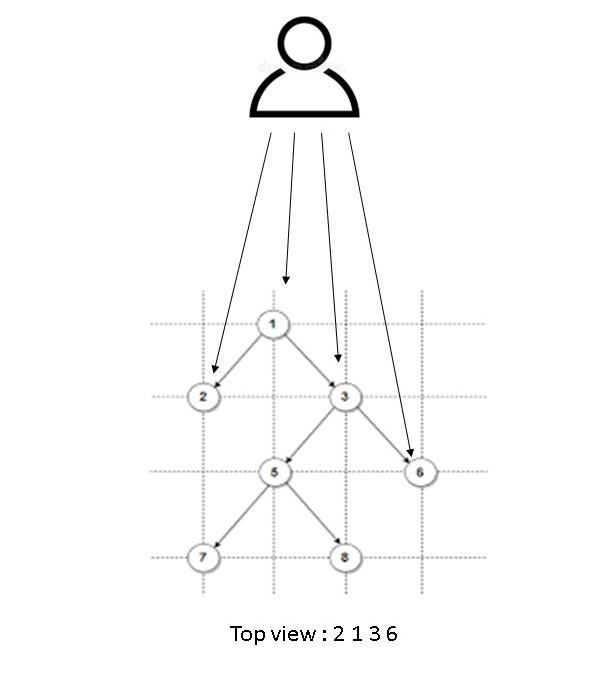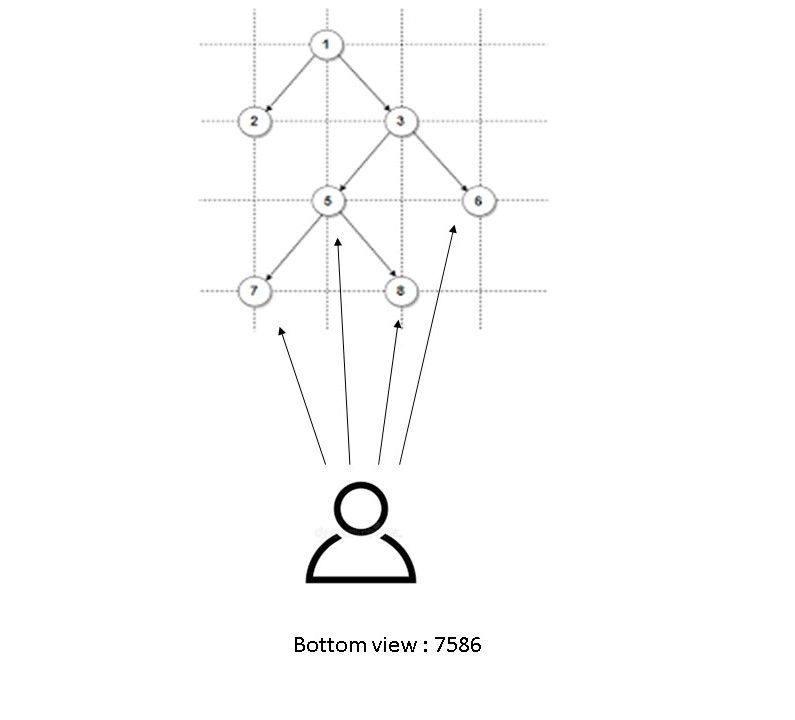×

Search anything:

# Types of views in Binary tree

#### Algorithms Problems on Binary TreeGet this book -> Problems on Array: For Interviews and Competitive Programming

In this article, we have explored the different types of views of the Binary Tree (like Left View) and discussed how to find them.

Binary tree: A tree whose elements have at most 2 children is called a binary tree. A Binary Tree node contains following parts- Data, Pointer to left child and Pointer to right child.

Different Types of Views in Binary Tree are :

• Left View
• Right View
• Top View
• Bottom View

Let us now discuss all of them one by one.

# Left View of Binary Tree

Left view of a Binary Tree is set of nodes visible when tree is viewed from left side.• Here we can clearly see our main task is to print the left most node of every level.
• We do a level order traversal on the tree and print the leftmost node at every level, which is also the first node of every level.
• To do this, we keep track of maximum level and traverse the tree in a manner where we visit the left subtree first and then the right subtree.
• Whenever we see a node whose level is more than maximum level so far, we print the node because this is the first node in its level.

Implementation:

``````#include<iostream>
using namespace std;

class  node{
public:
int data;
node* right;
node* left;
node(int d){
data=d;
left=NULL;
right=NULL;
}
};

void leftview(node *root,int level){

static int maxlevel=-1;
if(root==NULL){
return;
}
if(maxlevel<level){
cout<<root->data<<" ";
maxlevel=level;
}
leftview(root->left,level+1);
leftview(root->right,level+1);

}

int main() {

node* root = new node(1);
root->left = new node(2);
root->right = new node(3);
root->left->left = new node(4);
root->left->right = new node(5);
root->right->right = new node(6);

leftview(root,0);

}

``````

Input:

``````            1
/   \
2       3
/ \       \
4   5        6
``````

Output: 1 2 4

Explaination: When viewed from the left , we would see the nodes 1,2 and 4.

Time and Space complexity:

Time Complexity : O(n)
The function leftview does a simple traversal of the tree, so the time complexity is O(n).

Space Complexity :O(n)
Space required is O(n), due to the stack space during recursive call.

# Right View of Binary Tree

Right view of a Binary Tree is set of nodes visible when tree is viewed from Right side.• Here we can clearly see our main task is to print the right most node of every level.
• We do a level order traversal on the tree and print the rightmost node at every level, which is also the last node in every level.
• To do this, we keep track of maximum level and traverse the tree in a manner that right subtree is visited before left subtree.
• Whenever we see a node whose level is more than maximum level so far, we print the node because this is the last node in its level.

Implementation:

``````#include<iostream>
using namespace std;

class  node{
public:
int data;
node* right;
node* left;
node(int d){
data=d;
left=NULL;
right=NULL;
}
};

void rightview(node *root,int level){

static int maxlevel=-1;
if(root==NULL){
return;
}
if(maxlevel<level){
cout<<root->data<<" ";
maxlevel=level;
}

rightview(root->right,level+1);
rightview(root->left,level+1);

}

int main() {

node* root = new node(1);
root->left = new node(2);
root->right = new node(3);
root->left->left = new node(4);
root->left->right = new node(5);
root->right->right = new node(6);

rightview(root,0);

}
``````

Input:

``````             1
/     \
2          3
/     \         \
4       5          6
``````

Output: 1 3 6

Explaination: When viewed from the right , we would see the nodes 1,3 and 6.

Time and Space complexity:

Time Complexity : O(n)
The function righttview does a simple traversal of the tree, so the time complexity is O(n).

Space Complexity :O(n)
Space required is O(n), due to the stack space during recursive call.

# Top view of Binary Tree

Top view of a binary tree is the set of nodes visible when the tree is viewed from the top.• we create a empty map, where each key represents the relative horizontal distance of the node from the root node, and the value in the map maintains a pair containing the node’s value and its level number.
• Then we perform preorder traversal on the tree.
• If the current node’s level is less than the maximum level seen so far for the same horizontal distance as the current node or current horizontal distance is seen for the first time then we update the value and the level for the current horizontal distance in the map.
• For each node, while going for its left subtree we decrease the horizontal distance and increase the level by one, and for right subtree we increase both level and horizontal distance by one.

Implementation:

``````#include <iostream>
#include <map>
using namespace std;

struct node
{
int key;
node *left, *right;

node(int key)
{
this->key = key;
this->left = this->right = nullptr;
}
};

void topView(node* root, int dist, int level, map<int, pair<int, int>> &m)
{

if (root == nullptr) {
return;
}

if (m.find(dist) == m.end() || level < m[dist].second)
{
m[dist] = { root->key, level };
}

topView(root->left, dist - 1, level + 1, m);

topView(root->right, dist + 1, level + 1, m);
}

void topView(node* root)
{

map<int, pair<int, int>> m;

topView(root, 0, 0, m);

for (auto it: m) {
cout << it.second.first << " ";
}
}

int main()
{
node* root = new node(1);
root->left = new node(2);
root->right = new node(3);
root->left->left= new node(4);
root->left->right = new node(5);
root->right->left = new node(6);

topView(root);

return 0;
}

``````

Input:

``````             1
/      \
2           3
/     \       /
4       5     6
``````

Output: 4 2 1 3

Explaination: When viewed from the top , we would see the nodes 4 2 1 3.

Time and Space complexity:

Time Complexity: O(n log n)
Here we do a simple traversal of all the nodes in O (n). Along with this, each insertion in our map takes O (log n) time. So the Overall time Complexity will is O(n log n).

Space Complexity :O(n)
Stack space during recursive call is O(n) and also we require auxiliary space for the map. In the worst case, if the tree is skewed then the map will require O(n) space, therefore overall time complexity is O(n).

# Bottom View of Binary Tree

Bottom view of a binary tree is the set of nodes visible when the tree is viewed from the bottom.• We create an empty map where each key represents the relative horizontal distance of the node from the root node, and the value in the map maintains a pair containing the nodes value and its level number.
• Then we perform preorder traversal.
• If the current nodes level is more than or equal to the maximum level seen so far for the same horizontal distance as the current nodes or current horizontal distance is seen for the first time then we update the value and the level for the current horizontal distance in the map.
• For each node, while going to it's left subtree we decrease the horizontal distance and increasing level by one, and for right subtree we increase both level and horizontal distance by one.

Implementation:

``````#include <iostream>
#include <map>
using namespace std;

struct node
{
int key;
node *left, *right;

node(int key)
{
this->key = key;
this->left = this->right = nullptr;
}
};

void bottomView(node* node, int dist, int level, map<int, pair<int, int>> &map)
{

if (node == nullptr) {
return;
}

if (level >= map[dist].second)
{
map[dist] = { node->key, level };
}

bottomView(node->left, dist - 1, level + 1, map);

bottomView(node->right, dist + 1, level + 1, map);
}

void bottomView(node* root)
{
map<int, pair<int, int>> map;

bottomView(root, 0, 0, map);

for (auto it: map) {
cout << it.second.first << " ";
}
}

int main()
{
node* root = new node(1);
root->left = new node(2);
root->right = new node(3);
root->left->left= new node(4);
root->left->right = new node(5);
root->right->left = new node(6);

bottomView(root);

return 0;
}

``````

Input:

``````             1
/      \
2           3
/     \       /
4       5     6
``````

output: 4 2 6 3

Explaination: When viewed from the bottom , we would see the nodes 4 2 6 3.

Time and Space complexity:

Time complexity: O(n log n)
Here we do a simple traversal of all the nodes in O(n). Along with this, each insertion in our map takes O(log n) time. So the Overall time Complexity will is O(n log n).

Space Complexity :O(n)
Stack space during recursive call is O(n) and also we require auxiliary space for the map. In the worst case, if the tree is skewed then the map will require O(n) space, therefore overall time complexity is O(n).

With this article at OpenGenus, you must have the complete idea of the different views of a binary tree and how to find them.#### Shreya Shah

Intern at OpenGenus, B. Tech Student at Jabalpur Engineering College (JEC) in Information Technology, 2023.

Types of views in Binary tree